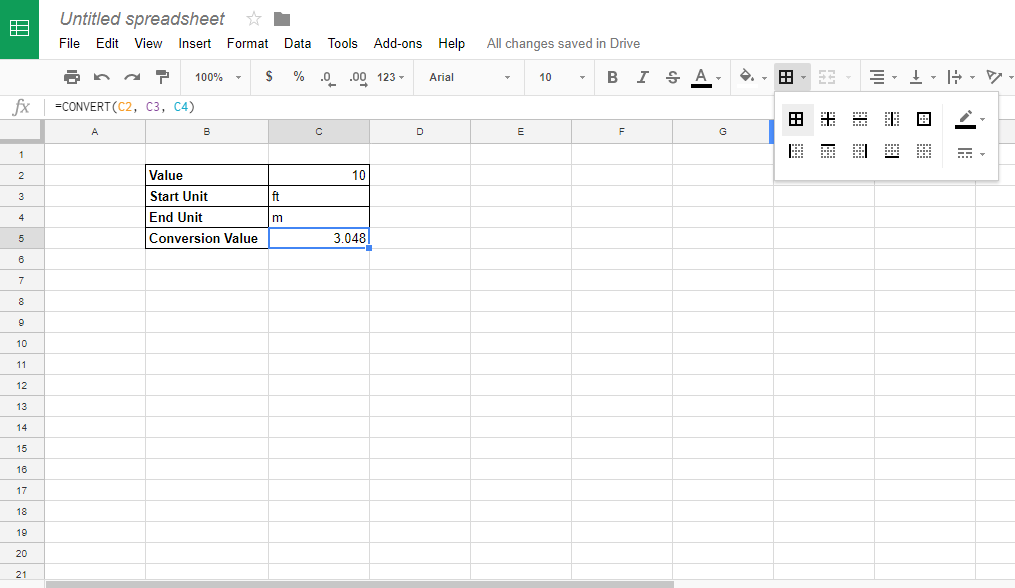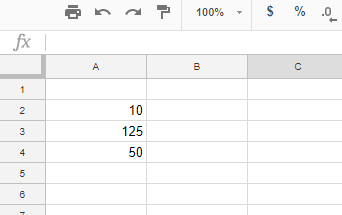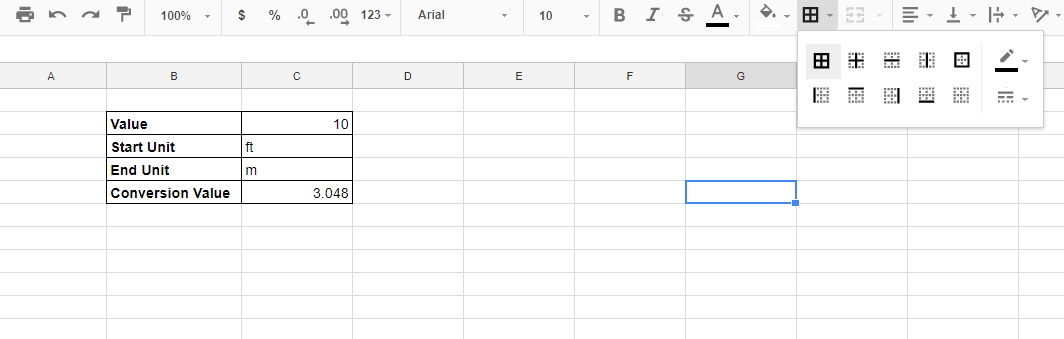# How To Convert Feet to Meters in Google Sheets

Many spreadsheet users need to convert numeric values to alternative measurement units. As such, most spreadsheet applications include a conversion function with which you can convert distance, area, volume, temperature and speed units among others. Google Sheets is no exception as it includes a CONVERT function. This is how you can convert feet values to meters in Google Sheets.## Convert Feet to Meters without CONVERT

Sheets’ CONVERSION function is not essential for converting measurement values. Instead, Google Sheets users can convert a feet value to meters with the actual conversion formula they would enter in a calculator. There are two basic formulas that convert feet to meters.

One meter amounts to 3.28 feet. Thus, the first way to convert feet values to meters is to divide a number by 3.28. For example, 10 feet amounts to 3.048 meters.

A foot amounts to approx 30.48 percent of a meter. Consequently, you can also multiply the value by 0.3048. Multiplying 10 feet by 0.3048 also returns 3.048.

Google Sheets users can convert feet to meters by entering the division (/) and multiplication (*) directly in the function bar. For an example, open a blank Google Sheets spreadsheet. Then enter ’10,’ ‘125’ and ’50’ in cells A2:A4 as in the snapshot directly below.Select cell B2, and enter ‘=A2/3.28’ in the fx bar. Cell B2 will return the value 3.048 when you press Enter. You can copy that formula to cells B3 and B4 by left-clicking the bottom right corner of B2 and holding the left mouse button. Drag the cursor over B3 and B4 copy the formula to those cells as shown in the snapshot below.Next, enter ‘=A2*30.48’ in cell C2. Cell C2 will then include 3.048, which is the same as B2. The difference is that C2’s value is rounded down to three decimal places. Copy C2 to C3 and C4 with the fill handle as you did for the B column.Now remove some decimal places from the B column’s values. You can remove decimal places by selecting a cell and pressing the Decrease decimal place button on Sheets’ toolbar. Alternatively, press the Increase decimal place button to add more decimals to a selected number. Your spreadsheet will match the one in the shot below when all the B and C column numbers have two decimal places.## The CONVERT Function

CONVERT is Sheets’ function for converting units of measure. Google Sheets users can enter this function in their spreadsheets with the syntax: CONVERT(value, start_unit, end_unit). The value can be a cell reference or a number included within the function. To convert feet values to meters, include feet (ft) as the start unit and meters (m) as the end unit.

Add CONVERT to your spreadsheet by selecting cell D2. Enter ‘=CONVERT(A2, “ft”, “m”)’ in the fx bar, and press the Return key. Cell D2 will now include the result 3.048, which you can round down to 3.05 as below.## Set up a Conversion Calculator

You can set up your very own conversion calculator with CONVERT. To do that, click File > New in Sheets to open a blank spreadsheet. Enter ‘Value,’ ‘Start Unit,’ ‘End Unit’ and ‘Conversion Value’ in cells B2:B5 as in the snapshot directly below.Next, select cell C5 and enter ‘=CONVERT(C2, C3, C4)’ in the fx bar. Enter ’10’ in cell C2, which is the feet value. Then you can enter ‘ft’ in C3 as the start unit, and input ‘m’ in cell C4 as the end unit. Cell C5 will now include 3.048 as the conversion value.As the conversion calculator has start and end unit cells, you can also convert other units of measure with it. For example, enter ‘yd’ in C3 and ‘ft’ in C4 instead to convert 10 yards to feet. The Google Sheets CONVERT function can convert time, distance, energy, volume, area, speed, temperature and more besides.

Finally, add some formatting to the conversion calculator. Select the cells B2:B5 and press the Ctrl + B hotkey to add bold to the headings. To add cell borders to the conversion calculator, select the cell range B2:C5 with cursor. Press the Borders button, and click the full border option shown in the snapshot below.Now you have your very own conversion calculator to convert feet to meters and other measurements with! To convert feet to meters in an Excel spreadsheet, check out this Tech Junkie guide.

Disclaimer: Some pages on this site may include an affiliate link. This does not effect our editorial in any way.

Todays Highlights